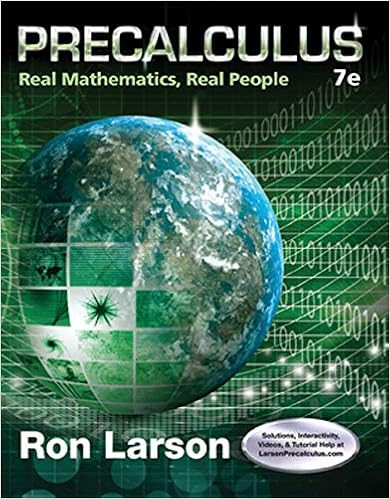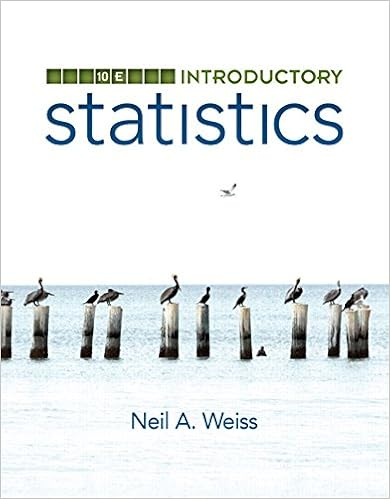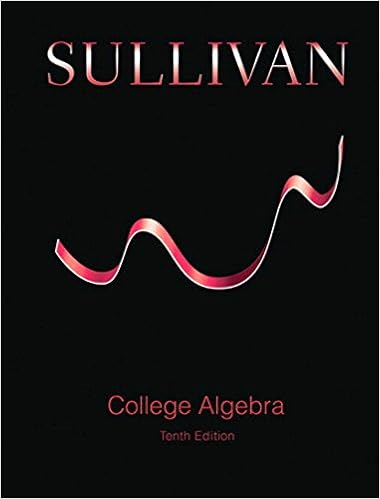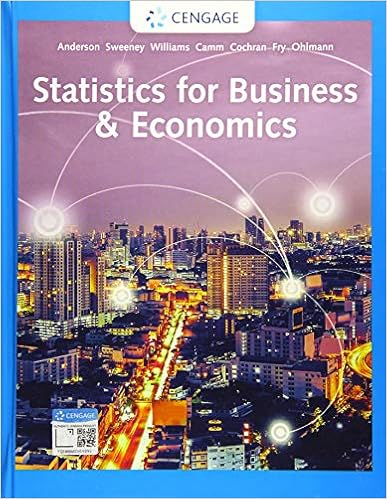# 482 a how much interest will he earn each year 483

• l2352566
• 28
• 71% (21) 15 out of 21 people found this document helpful

This preview shows page 27 - 28 out of 28 pages.

482. a) How much interest will he earn each year?
484. Part 2485. b) What will be the total value of the account after eight years?
487. Question488. Charlene invested \$4,000 at 6 1/2% simple interest for 5 years.489. Part 1490. a. How much interest will she earn in 5 years?
##### We have textbook solutions for you!The document you are viewing contains questions related to this textbook.
Chapter 3 / Exercise 05
Precalculus: Real Mathematics, Real People
LarsonExpert VerifiedBrowse all Textbook Solutions
493. Part 2494. b. What will be the total value of the account after 5 years?
496. Question497. Now let's compare interest compounded continuously to simple interest. Suppose that\$80,000 is invested in two accounts: one earns 4% simple interest, the other earns 4%compounded continuously. Fill in the following tables with the future value of eachaccount after each term. Use the formula A = Pe^rt for interest compoundedcontinuously. Round your answers to the nearest cent.498. Question499. An investor has \$20,000 to invest and is willing to keep it invested for up to five years. Youinvest \$20,000 in a savings account that offers 1.63% compounded monthly. You canremove the money any time you like, but plan to keep it in the investment for 5 years. Donot round intermediate calculations.500. Part 1501. What is the future value of the investment? Round your answer to the nearest cent.502. The future value of this investment is \$ .503. Answer504. Part 2505. What is the effective rate? Round your answer to two decimal places.
End of preview. Want to read all 28 pages?

Course Hero member to access this document

Term
Fall
Professor
Temkin
Tags
##### We have textbook solutions for you!
The document you are viewing contains questions related to this textbook.The document you are viewing contains questions related to this textbook.
Chapter 3 / Exercise 05
Precalculus: Real Mathematics, Real People
LarsonExpert Verified
•••